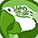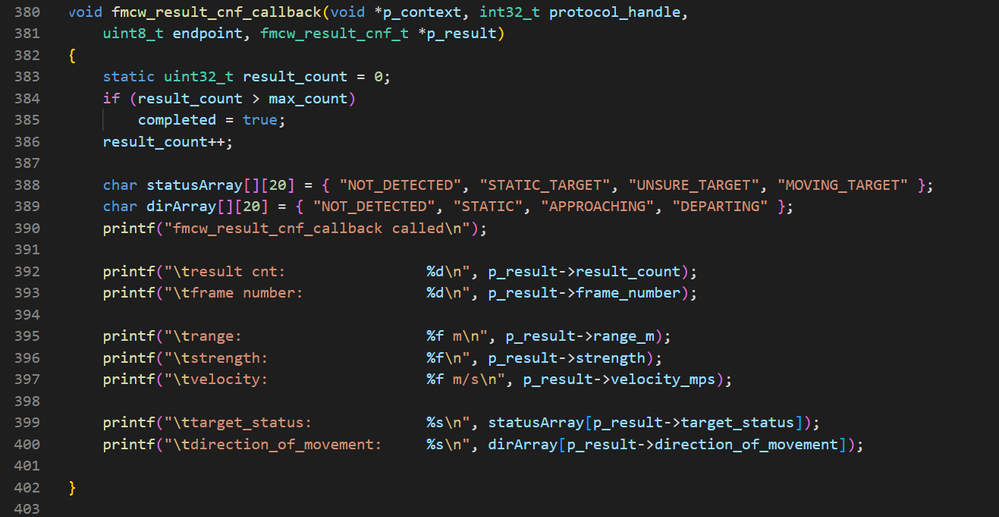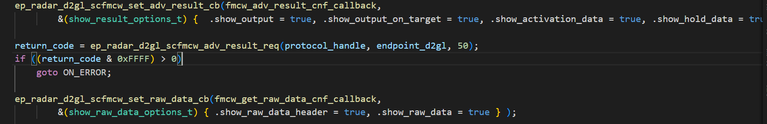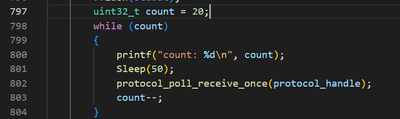Level 1Level 1

# Obtain distance from raw data [D2GL]

Hello!

Im using Distance2GoL (Software-Controlled FMCW radar)

I am trying to obtain the distance parameter when running the example C-Code. However the only printed output doesnt seem to be sufficient to calcualte the distance. And i cant find the distance elsewhere in the code aswell.

The printed data aswell as the corresponding part in the in code is attached. The code is commlib24_D2GL_basics.c line 555.

"Sample-Data" seems to be a float between 0 and 1 but im not quite sure what that represent. When im trying to use the formular for the distance R in the documentation im missing the variable "fb (beat frequency corresponding to the target).

Is it even possible to extract the raw distance data when using the c-code? I am planing to use the distance as a live parameter for a sound project.

Thanks alot!

luca

2 RepliesModeratorModerator

# Re: Obtain distance from raw data [D2GL]

Hello Luca,

1) The example C-Code of Distance2GoL is having functions of raw data and result of detected target called separately in the main code. The Raw data function fmcw_get_raw_data_cnf_callback prints frame number, chirp number, receiver number, ADC number, number of samples, sample data respectively.

Whereas fmcw_adv_result_cnf_callback function has range or distance, strength, velocity, target status, direction of movement which can be visible later, if you can run the code for more than 2-3 counts.

2)The function in the above snippet has the range parameter which gives distance of the target.

3) Sample_Data is the data from ADC in the normalized form which will be a float value between 0 and 1.

4) The beat frequency can be obtained using a Fast Fourier Transform(FFT) on Intermediate Frequency (IF)signal to find the distance R, but it is not required for you to do this because Distance2GoL displays the distance as mentioned above.You can also skip printing the raw data by making  .show_raw_data = false and .show_raw_data_header = false

5) If you want to print the range/distance as a live parameter, you can remove count and run the while loop continuously.Thanks and Regards,

PugithaLevel 1Level 1

# Re: Obtain distance from raw data [D2GL]

Hey Pugitha,

thanks a lot for your answer. I managed to print the distance in a loop to the console, independent from the count variable by setting the loop to while(true). So far it's working but now the loop breaks after 100 iterations, which I set as an argument (repeat_count) in the function call in line 785 and 792. Is it possible to run the loop infinitly?

best regards

luca